# Data Analysis and Visualization Using R: Lesson 2

David Robinson
1/29/14

## Introduction to ggplot2

`ggplot2` is a third party package that produces attractive visualizations of data easily and intuitively.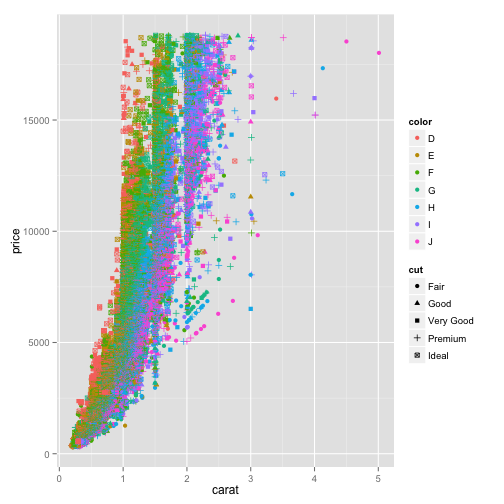### Installing ggplot2

`ggplot2` is a third party package: code that doesn't come built in to R. You therefore have to install it. The easiest way is to run the line:

``````install.packages("ggplot2")
``````

You can also go to the Tools->Install Packages… menu in RStudio.

Every time you reopen R, you need to load a library using `library()` before using it:

``````library(ggplot2)
``````

### Diamond data

``````data(diamonds)
``````

Contains information on the weight, price, size and quality of ~54,000 diamonds.

``````head(diamonds, 5)
``````
``````  carat     cut color clarity depth table price    x
1  0.23   Ideal     E     SI2  61.5    55   326 3.95
2  0.21 Premium     E     SI1  59.8    61   326 3.89
3  0.23    Good     E     VS1  56.9    65   327 4.05
4  0.29 Premium     I     VS2  62.4    58   334 4.20
5  0.31    Good     J     SI2  63.3    58   335 4.34
y    z
1 3.98 2.43
2 3.84 2.31
3 4.07 2.31
4 4.23 2.63
5 4.35 2.75
``````

Some columns of the diamond data

``````head(diamonds\$cut)
``````
`````` Ideal     Premium   Good      Premium   Good
 Very Good
5 Levels: Fair < Good < Very Good < ... < Ideal
``````
``````head(diamonds\$color)
``````
`````` E E E I J J
Levels: D < E < F < G < H < I < J
``````

### Aesthetics

An aesthetic is one attribute that we can perceive visually. For a scatter plot, some aesthetics are:

• x
• y
• color
• size
• shape

### ggplot call

To build a plot in ggplot2, we use four components:

`ggplot(`data`,`aesthetics`) + geom_`type of graph`() +` extra options

• data: The data frame we're working from
• aesthetics: which attributes (columns) of the data are represented by what visual qualities (x, y, color, size, shape…)
• type of graph: `geom_point`, `geom_histogram`, `geom_boxplot`
• extra options: custom title or axis labels, background color, whether to make axes on log scale…

### Basic scatter plot

``````ggplot(diamonds, aes(x=carat, y=price)) + geom_point()
``````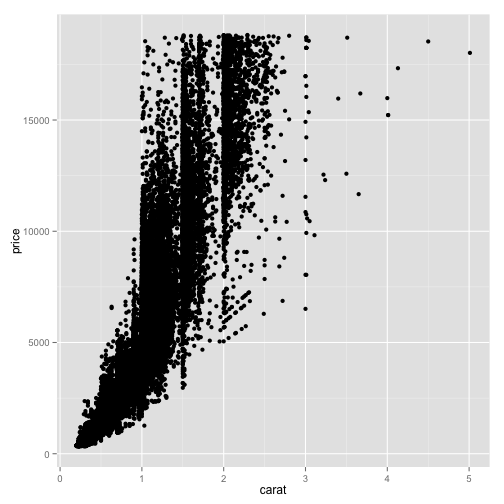``````ggplot(diamonds, aes(x=carat, y=price, color=color)) + geom_point()
``````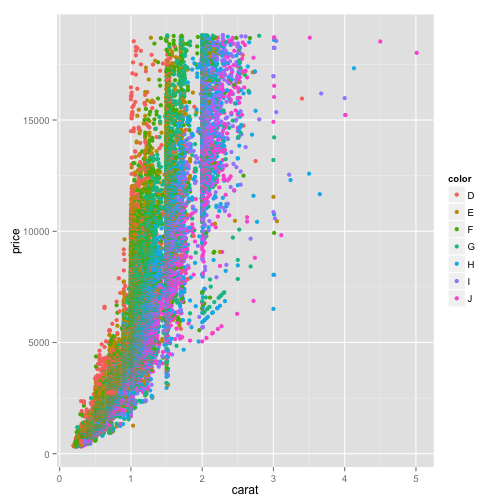``````ggplot(diamonds, aes(x=carat, y=price, color=color, shape=cut)) + geom_point()
````````````ggplot(diamonds, aes(x=carat, y=price, color=color, shape=cut, size=depth)) + geom_point()
``````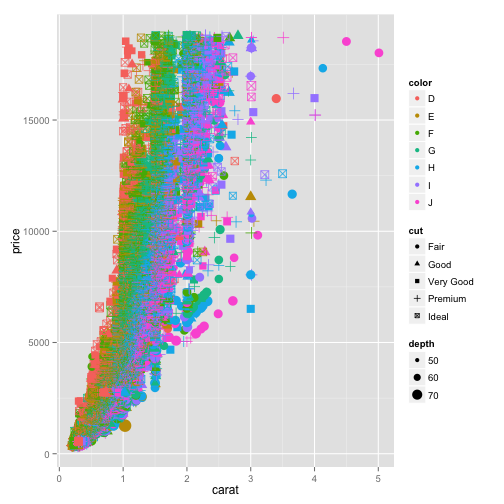### Plotting a subset of the dataset

``````ggplot(diamonds[1:100, ], aes(x=carat, y=price, color=clarity, shape=cut)) + geom_point()
``````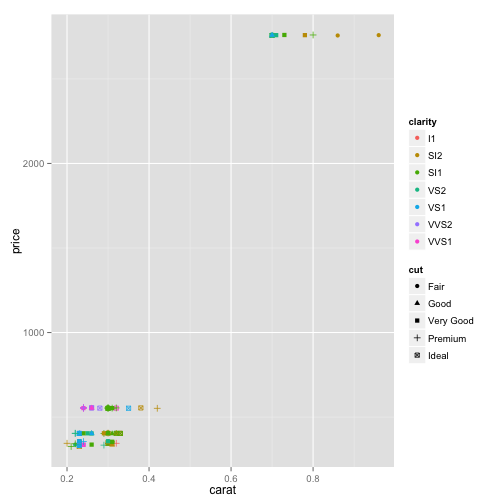### Pre-filtering the data frame based on one column

``````ggplot(diamonds[diamonds\$carat < 2, ], aes(x=carat, y=price, color=clarity, shape=cut)) + geom_point()
``````